Lessons learned: recursion, memoization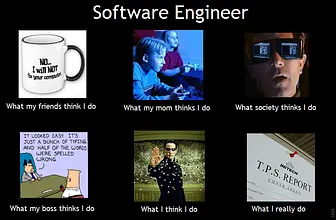Throughout my four years learning and experiencing Computer Science, I learned a lot of lessons along the way. From writing my first “Hello World…Lessons Learned: Arrays, binary search, recursion
Lesson: Array Manipulation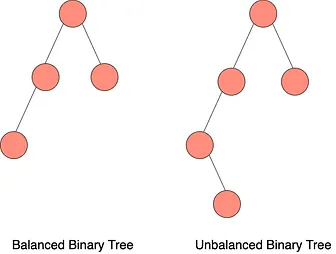Lessons Learned: Binary trees, recursion, error values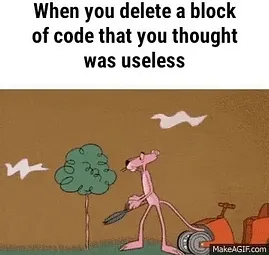Question: Given a string s and a dictionary of words dict, determine if s can be segmented into a space-separated sequence of one or more dictionary…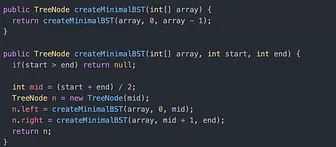Lessons Learned: Binary Search Tree, recursion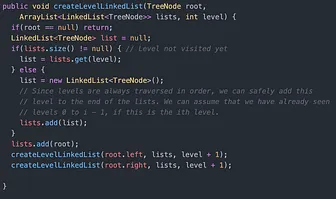Lessons Learned: recursion, tree traversalLessons Learned: stacks, sortingTime complexityQuestion 8: T1 and T2 are two very large binary trees, with T1 much bigger than T2. Create an algorithm to determine if T2 is a subtree of T1. One way…Question: Given a string containing just the characters ’(’, ’)’, ’’, ’’, ’[’ and ’]’, determine if the input string is valid.